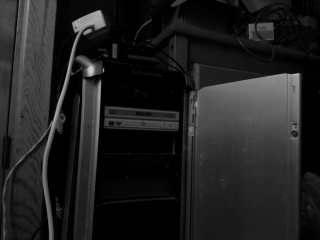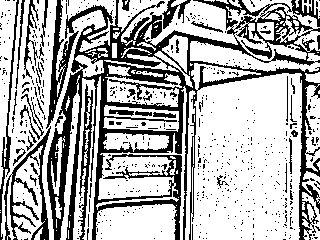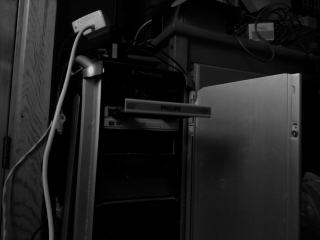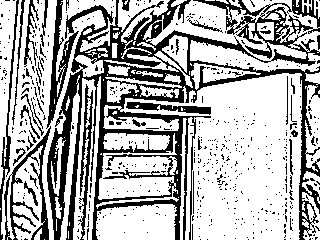# CDitter – CD-ROM drive based data exfiltration

We present a method for the exfiltration of data through the movement of a CD-ROM drive. The movement
of such drive is monitored through the use of a Raspberry Pi and camera. We make use of manchester encoding
to represent data. See https://en.wikipedia.org/wiki/Manchester_code for more
information.

## Grayscale and processed images## emit.py – Transmits data through the medium of a CD-ROM drive

We make use of a 4 second delay for both the closed & open CD-Drive state, potentially a lower value could be used, but I was keen
to avoid damaging the drive

```#!/usr/bin/python
import os
import time

os.system("eject -t")
time.sleep(15)
os.system("eject -T")
time.sleep(15)
os.system("eject -t")
time.sleep(15)

prev = 0

def emit(str):
global prev

for b in str:
for i in range(0,8):
bit = (ord(b) >> i) & 1

if bit == 0:
manc = [0,1]
else:
manc = [1,0]

for bv in manc:
print(bv)
if bv == 1:
if not prev == 1:
os.system("eject -T")
time.sleep(4)
else:
os.system("eject -t")
time.sleep(4)
prev = bv

emit("AZhello")
```

## cditter.py – capture and decode the output from the CD-ROM drive

```#!/usr/bin/python
import math
from picamera.array import PiRGBArray
from picamera import PiCamera
import time
import cv2
import numpy as np
from itertools import tee

def window(iterable, size):
iters = tee(iterable, size)
for i in range(1, size):
for each in iters[i:]:
next(each, None)
return zip(*iters)

def emit(str):
global prev
out = []
for b in str:
for i in range(0,8):
bit = (ord(b) >> i) & 1

if bit == 0:
out.append(0)
out.append(1)
else:
out.append(1)
out.append(0)
return out

preamble = emit("AZ")

def process(x):
m = [sum(y) / len(y) for y in zip(*x)]

for i in range(0,len(x)):
v1=x[i:len(x)]
new = []
for v in v1:
if v > m:
new.append(v)
new.append(v)
else:
new.append(v)
if new[0:len(preamble)] == preamble:
mdec = mancdec(new)
print("Data: ",tobytes(mdec[0:len(mdec) - (len(mdec) % 8)]))
break
def binary(arr):
m = 0
s = 0
for o in arr:
s = s +(o * (2**m))
m = m + 1
return s

def mancdec(arr):
out = []
for i in range(0,len(arr),2):
if arr[i:i+2] == [0,1]:
out.append(0)
elif arr[i:i+2] == [1,0]:
out.append(1)
return out

def tobytes(arr):
out = ""
for i in range(0,len(arr),8):
bits = arr[i:i+8]
out += chr(binary(bits))
return out

camera = PiCamera()
camera.resolution = (320, 240)
camera.framerate = 30
rawCapture = PiRGBArray(camera, size=camera.resolution)

# allow the camera to warmup
time.sleep(0.1)

bits = []

# from http://www.pyimagesearch.com/2014/09/15/python-compare-two-images/
def mse(imageA, imageB):
# the 'Mean Squared Error' between the two images is the
# sum of the squared difference between the two images;
# NOTE: the two images must have the same dimension
err = np.sum((imageA.astype("float") - imageB.astype("float")) ** 2)
err /= float(imageA.shape * imageA.shape)

# return the MSE, the lower the error, the more "similar"
# the two images are
return err

def extract(data):
out = []
x = [0,0]
c = 0
old = None

for d in data:
c += 1
x[d] += 1
if (not old == None and not d == old) or c == len(data):
if c == len(data):
out.append((d,x[d]))
else:
out.append((d^1,x[d^1]))
x[d^1] = 0
old = d

nout = []
for o in out:
if o > 3:
nout.append(o)

return nout

old = None
trained = False

imgl = []
trainv = []

for frame in camera.capture_continuous(rawCapture, format="bgr", use_video_port=True):
image = frame.array
gray = cv2.cvtColor(image, cv2.COLOR_BGR2GRAY)

if not old == None and trained == False:
#print(chr(27) + "[2J")
print("Training...")
count = 0
simid = -1
simv = float("inf")

for img in imgl:
s = mse(gray,img)
if s < simv:
simid = count
simv = s

count += 1
print("Similarity: ",simv)
if simv > 2600:
imgl.append([gray,1])
simid = len(imgl)-1
else:
imgl[simid]+=1
imgl[simid] = gray

trainv = []
for i in range(0,len(imgl)):
if imgl[i] > 20:
trainv.append([imgl[i],imgl[i]])
if len(trainv) == 2:
break

if len(trainv) == 2:
print("Trained...")
trained = True

elif trained:
simid = -1
simv = float("inf")
count = 0
for img in trainv:
s = mse(gray,img)
if s < simv:
simid = count
simv = s
count += 1

trainv[simid] = gray
trainv[simid] += 1
print(chr(27) + "[2J")
print("Current bit: ",simid)

bits.append(simid)
d = extract(bits)
if len(d) > 8:
process(d)
else:
imgl.append([gray,1])

old = image
rawCapture.truncate(0)
```

## Raspberry Pi

The following libraries are needed for the program to run

```sudo apt-get update
sudo apt-get install python-opencv
sudo apt-get install python-pip
sudo pip install picamera
sudo pip install numpy
```

## Improvements

I’m sure the time to transmit data could be substantially reduced by decreasing the delays used for the CD tray eject/close commands and also possibly using an alternative technique to manchester encoding.

Additionally the initial 15 second delays used for the training period could likely be reduced.

It might be nice also to properly packetise the data like so:

|preamble|packet length|packet contents|

At the moment I’ve only tested with a single preamble with data following it